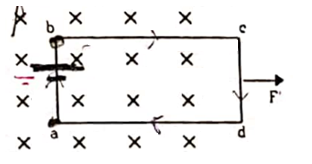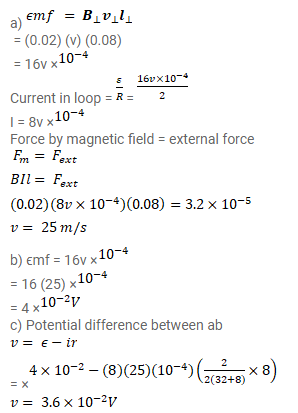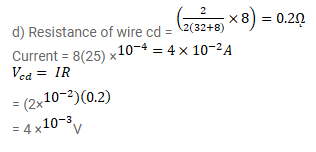# A rectangular frame of wire abcd has dimensions

Question:

A rectangular frame of wire abcd has dimensions $32 \mathrm{~cm} \times 8.0 \mathrm{~cm}$ and a total resistance of $2.0 \Omega$. It is pulled out of a magnetic field $B=0.020 \mathrm{~T}$ by applying a force of $3.2 \times 10^{-5} \mathrm{~N}$. It is found that the frame moves with constant speed. Find

(a) this constant speed,

(b) the emf induced in the loop,

(c) the potential difference between the points a and b and

(d) the potential difference between the points $c$ and $d$.Solution: